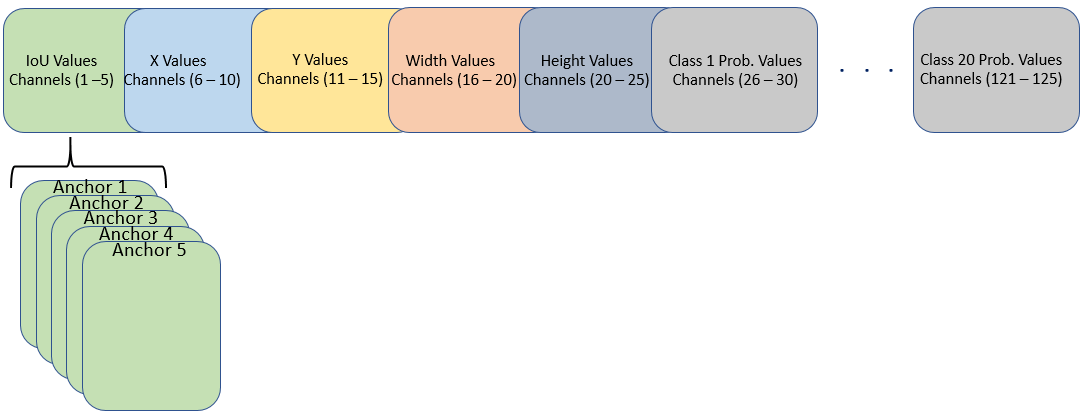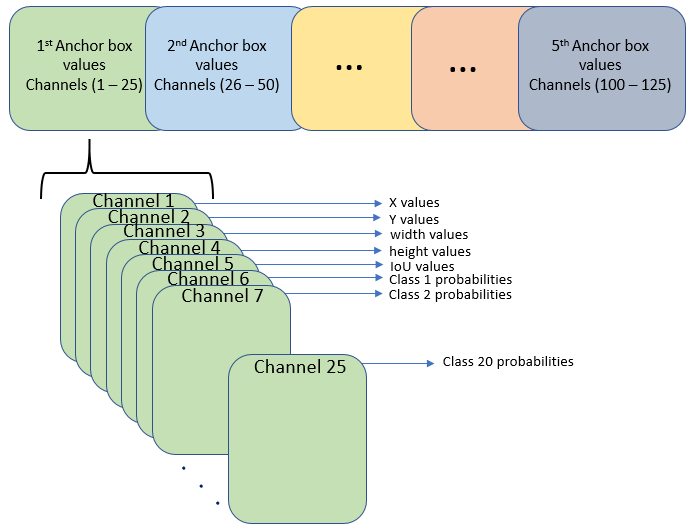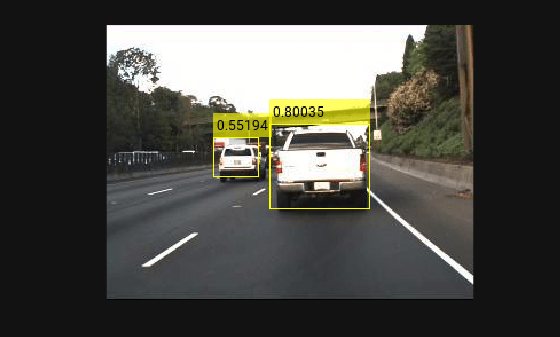# Import Pretrained ONNX YOLO v2 Object Detector

This example shows how to import a pretrained ONNX™ (Open Neural Network Exchange) you only look once (YOLO) v2  object detection network and use it to detect objects. After you import the network, you can deploy it to embedded platforms using GPU Coder™ or retrain it on custom data using transfer learning with `trainYOLOv2ObjectDetector`.

```pretrainedURL = 'https://ssd.mathworks.com/supportfiles/vision/deeplearning/models/yolov2/tiny_yolov2.tar'; pretrainedNetTar = 'yolov2Tiny.tar'; if ~exist(pretrainedNetTar,'file') disp('Downloading pretrained network (58 MB)...'); websave(pretrainedNetTar,pretrainedURL); end```

### Extract YOLO v2 Network

Untar the downloaded file to extract the Tiny YOLO v2 network. Load the `'Model.onnx'` model from `tiny_yolov2` folder, which is an ONNX YOLO v2 network pretrained on the PASCAL VOC data set . The network can detect objects from 20 different classes .

```onnxfiles = untar(pretrainedNetTar); pretrainedNet = fullfile('tiny_yolov2','Model.onnx');```

### Import ONNX YOLO v2 Layers

Use the `importONNXLayers` function to import the downloaded network.

`lgraph = importONNXLayers(pretrainedNet,'ImportWeights',true);`

importONNXLayers adds regression layer at the end by default. Remove the last regression layer added by importONNXLayers as `yolov2ObjectDetector` expects YOLO v2 detection network to end with `yolov2OutputLayer`. For more information on YOLO v2 detection network, see Getting Started with YOLO v2 (Computer Vision Toolbox).

`lgraph = removeLayers(lgraph,'RegressionLayer_grid');`

The Add YOLO v2 Transform and Output Layers section shows how to add YOLO v2 output layer along with YOLO v2 Transform layer to the imported layers.

The network in this example contains no unsupported layers. Note that if the network you want to import has unsupported layers, the function imports them as placeholder layers. Before you can use your imported network, you must replace these layers. For more information on replacing placeholder layers, see `findPlaceholderLayers`.

### Define YOLO v2 Anchor Boxes

YOLO v2 uses predefined anchor boxes to predict object location. The anchor boxes used in the imported network are defined in the Tiny YOLO v2 network configuration file . The ONNX anchors are defined with respect to the output size of the final convolution layer, which is 13-by-13. To use the anchors with `yolov2ObjectDetector`, resize the anchor boxes to the network input size, which is 416-by-416. The anchor boxes for `yolov2ObjectDetector` must be specified in the form [height, width].

```onnxAnchors = [1.08,1.19; 3.42,4.41; 6.63,11.38; 9.42,5.11; 16.62,10.52]; inputSize = lgraph.Layers(1,1).InputSize(1:2); lastActivationSize = [13,13]; upScaleFactor = inputSize./lastActivationSize; anchorBoxesTmp = upScaleFactor.* onnxAnchors; anchorBoxes = [anchorBoxesTmp(:,2),anchorBoxesTmp(:,1)];```

### Reorder Detection Layer Weights

For efficient processing, you must reorder the weights and biases of the last convolution layer in the imported network to obtain the activations in the arrangement that `yolov2ObjectDetector` requires. `yolov2ObjectDetector` expects the 125 channels of the feature map of the last convolution layer in the following arrangement:

• Channels 1 to 5 - IoU values for five anchors

• Channels 6 to 10 - X values for five anchors

• Channels 11 to 15 - Y values for five anchors

• Channels 16 to 20 - Width values for five anchors

• Channels 21 to 25 - Height values for five anchors

• Channels 26 to 30 - Class 1 probability values for five anchors

• Channels 31 to 35 - Class 2 probability values for five anchors

• Channels 121 to 125 - Class 20 probability values for five anchorsHowever, in the last convolution layer, which is of size 13-by-13, the activations are arranged differently. Each of the 25 channels in the feature map corresponds to:

• Channel 1 - X values

• Channel 2 - Y values

• Channel 3 - Width values

• Channel 4 - Height values

• Channel 5 - IoU values

• Channel 6 - Class 1 probability values

• Channel 7 - Class 2 probability values

• Channel 25 - Class 20 probability valuesUse the supporting function `rearrangeONNXWeights`, listed at the end of this example, to reorder the weights and biases of the last convolution layer in the imported network and obtain the activations in the format required by `yolov2ObjectDetector`.

```weights = lgraph.Layers(end,1).Weights; bias = lgraph.Layers(end,1).Bias; layerName = lgraph.Layers(end,1).Name; numAnchorBoxes = size(onnxAnchors,1); [modWeights,modBias] = rearrangeONNXWeights(weights,bias,numAnchorBoxes);```

Replace the weights and biases of the last convolution layer in the imported network with the new convolution layer using the reordered weights and biases.

```filterSize = size(modWeights,[1 2]); numFilters = size(modWeights,4); modConvolution8 = convolution2dLayer(filterSize,numFilters,... 'Name',layerName,'Bias',modBias,'Weights',modWeights); lgraph = replaceLayer(lgraph,'convolution8',modConvolution8);```

### Add YOLO v2 Transform and Output Layers

A YOLO v2 detection network requires the YOLO v2 transform and YOLO v2 output layers. Create both of these layers, stack them in series, and attach the YOLO v2 transform layer to the last convolution layer.

```classNames = tinyYOLOv2Classes; layersToAdd = [ yolov2TransformLayer(numAnchorBoxes,'Name','yolov2Transform'); yolov2OutputLayer(anchorBoxes,'Classes',classNames,'Name','yolov2Output'); ]; lgraph = addLayers(lgraph, layersToAdd); lgraph = connectLayers(lgraph,layerName,'yolov2Transform');```

The `ElementwiseAffineLayer `in the imported network duplicates the preprocessing step performed by `yolov2ObjectDetector`. Hence, remove the `ElementwiseAffineLayer` from the imported network.

```yoloScaleLayerIdx = find(... arrayfun( @(x)isa(x,'nnet.onnx.layer.ElementwiseAffineLayer'), ... lgraph.Layers)); if ~isempty(yoloScaleLayerIdx) for i = 1:size(yoloScaleLayerIdx,1) layerNames {i} = lgraph.Layers(yoloScaleLayerIdx(i,1),1).Name; end lgraph = removeLayers(lgraph,layerNames); lgraph = connectLayers(lgraph,'image','convolution'); end```

### Create YOLO v2 Object Detector

Assemble the layer graph using the `assembleNetwork` function and create a YOLO v2 object detector using the `yolov2ObjectDetector` function.

`net = assembleNetwork(lgraph)`
```net = DAGNetwork with properties: Layers: [34×1 nnet.cnn.layer.Layer] Connections: [33×2 table] InputNames: {'image'} OutputNames: {'yolov2Output'} ```
`yolov2Detector = yolov2ObjectDetector(net)`
```yolov2Detector = yolov2ObjectDetector with properties: ModelName: 'importedNetwork' Network: [1×1 DAGNetwork] TrainingImageSize: [416 416] AnchorBoxes: [5×2 double] ClassNames: [aeroplane bicycle bird boat bottle bus car cat chair cow diningtable dog horse motorbike person pottedplant sheep sofa train tvmonitor] ```

### Detect Objects Using Imported YOLO v2 Detector

Use the imported detector to detect objects in a test image. Display the results.

```I = imread('highway.png'); % Convert image to BGR format. Ibgr = cat(3,I(:,:,3),I(:,:,2),I(:,:,1)); [bboxes, scores, labels] = detect(yolov2Detector, Ibgr); detectedImg = insertObjectAnnotation(I, 'rectangle', bboxes, scores); figure imshow(detectedImg);```### Supporting Functions

```function [modWeights,modBias] = rearrangeONNXWeights(weights,bias,numAnchorBoxes) %rearrangeONNXWeights rearranges the weights and biases of an imported YOLO %v2 network as required by yolov2ObjectDetector. numAnchorBoxes is a scalar %value containing the number of anchors that are used to reorder the weights and %biases. This function performs the following operations: % * Extract the weights and biases related to IoU, boxes, and classes. % * Reorder the extracted weights and biases as expected by yolov2ObjectDetector. % * Combine and reshape them back to the original dimensions. weightsSize = size(weights); biasSize = size(bias); sizeOfPredictions = biasSize(3)/numAnchorBoxes; % Reshape the weights with regard to the size of the predictions and anchors. reshapedWeights = reshape(weights,prod(weightsSize(1:3)),sizeOfPredictions,numAnchorBoxes); % Extract the weights related to IoU, boxes, and classes. weightsIou = reshapedWeights(:,5,:); weightsBoxes = reshapedWeights(:,1:4,:); weightsClasses = reshapedWeights(:,6:end,:); % Combine the weights of the extracted parameters as required by % yolov2ObjectDetector. reorderedWeights = cat(2,weightsIou,weightsBoxes,weightsClasses); permutedWeights = permute(reorderedWeights,[1 3 2]); % Reshape the new weights to the original size. modWeights = reshape(permutedWeights,weightsSize); % Reshape the biases with regared to the size of the predictions and anchors. reshapedBias = reshape(bias,sizeOfPredictions,numAnchorBoxes); % Extract the biases related to IoU, boxes, and classes. biasIou = reshapedBias(5,:); biasBoxes = reshapedBias(1:4,:); biasClasses = reshapedBias(6:end,:); % Combine the biases of the extracted parameters as required by yolov2ObjectDetector. reorderedBias = cat(1,biasIou,biasBoxes,biasClasses); permutedBias = permute(reorderedBias,[2 1]); % Reshape the new biases to the original size. modBias = reshape(permutedBias,biasSize); end function classes = tinyYOLOv2Classes() % Return the class names corresponding to the pretrained ONNX tiny YOLO v2 % network. % % The tiny YOLO v2 network is pretrained on the Pascal VOC data set, % which contains images from 20 different classes. classes = [ ... " aeroplane", "bicycle", "bird", "boat", "bottle", "bus", "car",... "cat", "chair", "cow", "diningtable", "dog", "horse", "motorbike",... "person", "pottedplant", "sheep", "sofa", "train", "tvmonitor"]; end```

### References

 Redmon, Joseph, and Ali Farhadi. "YOLO9000: Better, Faster, Stronger." In 2017 IEEE Conference on Computer Vision and Pattern Recognition (CVPR), 6517-25. Honolulu, HI: IEEE, 2017. https://doi.org/10.1109/CVPR.2017.690.